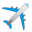Welcome to Microsoft Flight Simulator’s SDK Q&A Platform!

You have questions regarding the SDK? DevMode Tools? SimConnect? You would like to submit an idea for future improvements, seek help or exchange knowledge? You’re in the right place.

Please take a moment to read the platform’s guidelines before you get started!

# question

## Contact point static compression coefficient

In the FSX SDK, this variable was named simply "Static Compression." It's description stated, "This is the distance a landing gear is compressed when the empty aircraft is at rest on the ground (feet). This term defines the “strength” of the strut, where a smaller number will increase the “stiffness” of the strut."

In the MSFS SDK, this variable is described as "The static compression coefficient constant (which is used to compute spring reaction when on the ground), in ft. If the contact point is rigid, then set this to 0." This is confusing to me in that a coefficient is normally dimensionless. Does the FSX description still apply, that is, that this is the distance a landing gear is compressed when the empty aircraft is at rest on the ground (in feet)?

To follow on, the next variable was named the "Ratio of maximum compression to static compression" in the FSX SDK and was described as the ratio of the max dynamic compression available. In the MSFS SDK, this same variable is called the "maximum static compression ratio," which implies that it is the compression ratio of a stationary fully loaded airplane compared to an empty airplane, rather than, say, the compression of an airplane landing at a high ground loading compared to an empty static airplane. Can you clarify which is correct?

Up to 5 attachments (including images) can be used with a maximum of 4.8 MiB each and 23.8 MiB total.

No need to spend too much time on this right now. I am considering deleting the question. when I use IRL values for what I believe these variables represent, the resulting external view I get is not what I expect. For example, instead of the gear compressing, the bottom of the tire appears to go through the pavement giving a flattened look to the bottom of the tire.
0 Likes 0 ·

This is a great article that explains it for FSX and for all three of our aircraft so far in MSFS holds true.

https://www.fsdeveloper.com/wiki/index.php?title=Contact_Points

0 Likes 0 ·

·

The static compression coefficient constant is the distance in feet the contact point will compress when the aircraft is at a standstill and at max weight.

The maximum static compression ratio value times the static compression coefficient will be the maximum amount of available compression in feet.

So figure out how much total compression you will need, then determine what the resting compression distance should be, then use this formula to determine the max compression:
total compression / static compression coefficient = maximum static compression

When the suspension compresses, the entire 3D model will move. So in order for the wheels to remain on the ground, you need to animate the gear using frames 100-200. The wheels debugger in aircraft editor will help by showing you the distances in cm.

The last parameter in the contact points list is an exponential factor for the compression. You can try values between 1 and 2 to make the compression less linear.

The damping ratio constant controls how much oscillation the springs have. Not too high and not too low will work well.

Up to 5 attachments (including images) can be used with a maximum of 4.8 MiB each and 23.8 MiB total.

That seems to be quite a change from the FSX SDK, where the static compression variable was the static compression at an empty weight. Now the static compression coefficient variable is the compression at the max weight? That doesn't appear to match what I see when I look at the compression distance in the wheel debugger, but I may not be doing this correctly.

Since the force applied to a given contact point depends not only on the airplane weight, but also it's cg position, what is used for determining the static compression coefficient. Let's take a nose gear for example since there is only one of them to take all of the load. Let's further use a static compression coefficient of 1 ft (30.5 cm) and a maximum static compression ratio of 1.15. This would give a total (static) compression of 1.15 ft (35 cm).

When the airplane is empty (airplane total weight = OEW), and the cg is set to the forward limit, the wheel debug window gives a compression of 29/35 cm. This happens to be very close to the static compression coefficient. When the airplane is at the maximum takeoff weight, again at the forward cg limit, the debug window gives a compression of 35/35 cm. This is not the static compression coefficient amount; it is the total (static) compression. Even at the aft cg limit, the compression in the debug window is 33/35.

0 Likes 0 ·
I've only been able to determine these by testing many different values, so I could have made some errors.

I think with different values, you may see a more obvious result. In your example, there is very little travel (only 5 cm) and at rest, it already used up 85% of the travel. Since we cannot see the exact formulas used, it's difficult to determine the limits of the calculations.

My best results have been to have the static compression 30-40% of the total range.

0 Likes 0 ·